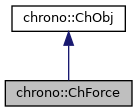chrono::ChForce Class Reference

## Description

Forces are objects which must be attached to rigid bodies in order to apply torque or force to such body.

ChForce objects are able to represent either forces and torques, depending on a flag.

#include <ChForce.h>

Inheritance diagram for chrono::ChForce:[legend]
Collaboration diagram for chrono::ChForce:[legend]

## Public Types

enum  ForceType { FORCE, TORQUE }
Types of force application.

enum  ReferenceFrame { BODY, WORLD }
Reference for position frame.

enum  AlignmentFrame { BODY_DIR, WORLD_DIR }
Reference for alignment.

## Public Member Functions

ChForce (const ChForce &other)

virtual ChForceClone () const override
"Virtual" copy constructor (covariant return type).

ChBodyGetBody ()
Return the parent body (the force belongs to this rigid body)

void SetBody (ChBody *newRB)
Sets the parent body (the force belongs to this rigid body)

void SetMode (ForceType m_mode)
Sets the mode (force or torque)

ForceType GetMode () const

void SetAlign (AlignmentFrame m_align)
Sets the alignment method. More...

AlignmentFrame GetAlign () const

void SetFrame (ReferenceFrame m_frame)
Sets the alignment method. More...

ReferenceFrame GetFrame () const

ChVector GetVpoint () const
Gets the application point, in absolute coordinates.

ChVector GetVrelpoint () const
Gets the application point, in rigid body coordinates.

void SetVpoint (ChVector<> mypoint)
Sets the application point, in absolute coordinates.

void SetVrelpoint (ChVector<> myrelpoint)
Sets the application point, in rigid body coordinates.

ChVector GetDir () const
Gets the force (or torque) direction, in absolute coordinates.

ChVector GetRelDir () const
Gets the force (or torque) direction, in rigid body coordinates.

void SetDir (ChVector<> newf)
Sets the force (or torque) direction, in absolute coordinates.

void SetRelDir (ChVector<> newf)
Sets the force (or torque) direction, in rigid body coordinates.

void SetMforce (double newf)
Sets force (or torque) modulus.

double GetMforce () const
Gets force (or torque) modulus.

void SetModulation (std::shared_ptr< ChFunction > m_funct)
Sets a function for time-modulation of the force.

std::shared_ptr< ChFunctionGetModulation () const

void SetMove_x (std::shared_ptr< ChFunction > m_funct)
Sets a function for time dependency of position (on x axis)

std::shared_ptr< ChFunctionGetMove_x () const

void SetMove_y (std::shared_ptr< ChFunction > m_funct)
Sets a function for time dependency of position (on y axis)

std::shared_ptr< ChFunctionGetMove_y () const

void SetMove_z (std::shared_ptr< ChFunction > m_funct)
Sets a function for time dependency of position (on z axis)

std::shared_ptr< ChFunctionGetMove_z () const

void SetF_x (std::shared_ptr< ChFunction > m_funct)
Sets a function for time dependency of force X component.

std::shared_ptr< ChFunctionGetF_x () const

void SetF_y (std::shared_ptr< ChFunction > m_funct)
Sets a function for time dependency of force Y component.

std::shared_ptr< ChFunctionGetF_y () const

void SetF_z (std::shared_ptr< ChFunction > m_funct)
Sets a function for time dependency of force Z component.

std::shared_ptr< ChFunctionGetF_z () const

ChVector GetForce () const
Gets the instant force vector -or torque vector- in absolute coordinates.

ChVector GetRelForce () const
Gets the instant force vector -or torque vector- in rigid body coordinates.

double GetForceMod () const
Gets the instant force vector -or torque vector- modulus.

const ChVectorN< double, 7 > & GetQf () const
Gets force-torque applied to rigid body, as lagrangian generalized force (7x1 matrix).

void GetBodyForceTorque (ChVector<> &body_force, ChVector<> &body_torque) const
Gets force-torque applied to rigid body, as force vector (in absol.coords) and torque vector (in body coords).

void UpdateTime (double mytime)

void Update (double mytime)

virtual void ArchiveOUT (ChArchiveOut &marchive) override
Method to allow serialization of transient data to archives.

virtual void ArchiveIN (ChArchiveIn &marchive) override
Method to allow deserialization of transient data from archives.Public Member Functions inherited from chrono::ChObj
ChObj (const ChObj &other)

int GetIdentifier () const
Gets the numerical identifier of the object.

void SetIdentifier (int id)
Sets the numerical identifier of the object.

double GetChTime () const
Gets the simulation time of this object.

void SetChTime (double m_time)
Sets the simulation time of this object.

const char * GetName () const
Gets the name of the object as C Ascii null-terminated string -for reading only!

void SetName (const char myname[])
Sets the name of this object, as ascii string.

std::string GetNameString () const
Gets the name of the object as C Ascii null-terminated string.

void SetNameString (const std::string &myname)
Sets the name of this object, as std::string.

void MFlagsSetAllOFF (int &mflag)

void MFlagsSetAllON (int &mflag)

void MFlagSetON (int &mflag, int mask)

void MFlagSetOFF (int &mflag, int mask)

int MFlagGet (int &mflag, int mask)

virtual std::string & ArchiveContainerName ()Protected Attributes inherited from chrono::ChObj
double ChTime
the time of simulation for the object

## ◆ SetAlign()

 void chrono::ChForce::SetAlign ( AlignmentFrame m_align )
inline

Sets the alignment method.

The force will rotate together with this reference.

## ◆ SetFrame()

 void chrono::ChForce::SetFrame ( ReferenceFrame m_frame )
inline

Sets the alignment method.

The force application point will follow this reference.

The documentation for this class was generated from the following files:
• /builds/uwsbel/chrono/src/chrono/physics/ChForce.h
• /builds/uwsbel/chrono/src/chrono/physics/ChForce.cpp